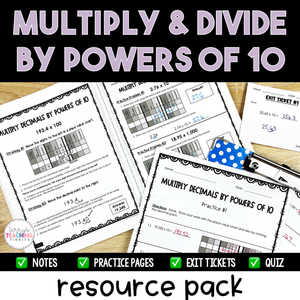# Multiply and Divide by Powers of 10 - Printable

• \$5.00
Unit price per
Shipping calculated at checkout.

Teach your students how to multiply and divide by powers of 10 using this comprehensive printable resource pack.  It has everything you need to teach this standard, including notes, practice, exit tickets, mixed practice, homework pages, and a quiz.

This resource begins with a mini-lesson on multiplying whole numbers by powers of ten.  I recommend using this mini-lesson at the beginning of the school year during your unit on whole numbers.  It’s a great opportunity to review place value from fourth grade while introducing powers of ten.  When you transition to decimals, you can then use the mini-lessons for multiplying decimals by powers of ten and dividing decimals by powers of ten.

This resource is complete, concise, and straightforward with a clear format.  It is low-prep and easy to assign to a sub.  If your students are struggling with place value, specifically multiplying and dividing by powers of ten,  this resource is for you!

Standard: 5.NBT.2

This resource is also part of my 5th Grade Math Year-Long Curriculum Bundle.

How Can You Use This?

Introduce each skill with the detailed set of notes and sample problems.  I suggest doing this in a whole group setting and pulling the PDF up on a Smartboard so students can annotate over the models.

Gradually release students to practice independently using the practice pages.  Check for understanding and mastery after teaching each skill using the exit tickets.

For additional practice, provide your students with the mixed practice pages for multiplying and dividing decimals by powers of 10, along with the homework pages for reinforcement at home.

When all skills have been taught, assess with the final quiz when you feel students are ready.

## What You Get

The Mini-Lessons Included:

• Multiplying Whole Numbers by Powers of Ten
• Multiplying Decimals by Powers of Ten
• Dividing Decimals by Powers of Ten

What You’ll Get in EACH Mini-lesson:

• Detailed Notes
• Practice Problems
• 2 Practice Activities
• 3 Exit Tickets

You’ll Also Get:

• 2 Mixed Review Pages (*Decimals Only*)
• 2 Homework Pages (*Decimals Only*)
• Quiz (*Decimals Only*)